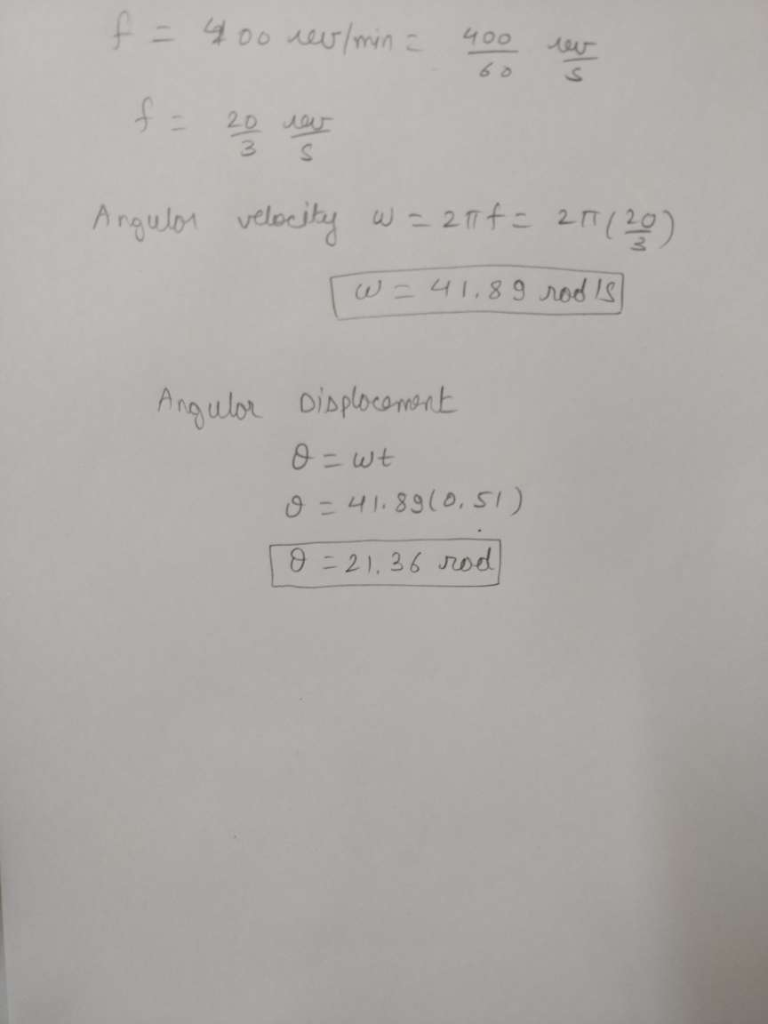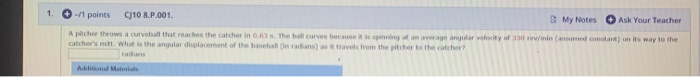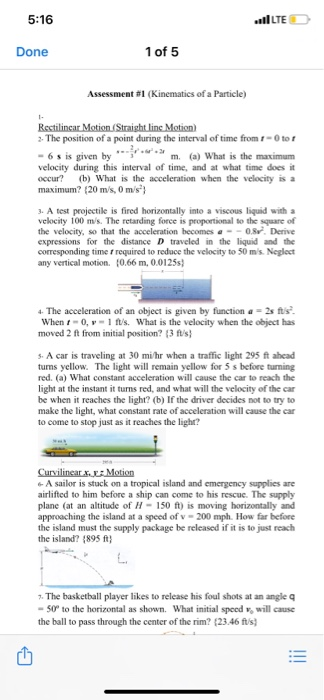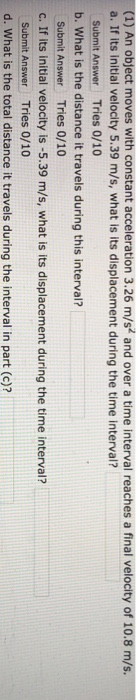# A pitcher throws a curveball that reaches the catcher in 0.51 s. The ball curves because...

A pitcher throws a curveball that reaches the catcher in 0.51 s. The ball curves because it is spinning at an average angular velocity of 400 rev/min (assumed constant) on its way to the catcher's mitt. What is the angular displacement of the baseball (in radians) as it travels from the pitcher to the catcher?#### Earn Coin

Coins can be redeemed for fabulous gifts.

Similar Homework Help Questions
• ### A pitcher throws a curveball that reaches the catcher in 0.70 s. The ball curves because...

A pitcher throws a curveball that reaches the catcher in 0.70 s. The ball curves because it is spinning at an average angular velocity of 370 rev/min (assumed constant) on its way to the catcher's mitt. What is the angular displacement of the baseball (in radians) as it travels from the pitcher to the catcher?

• ### A pitcher throws a curveball that reaches the catcher in 0.55 s

A pitcher throws a curveball that reaches the catcher in 0.55 s. The ball curves because it is spinning at an average angular velocity of 335 rev/min (assumed constant) on its way to the catcher's mitt. What is the angular displacement of the baseball (in radians) as it travels from the pitcher to the catcher? This depends upon the mass of the baseball, the orientation of ths spin axis ands the distance to home plate. Was any of this information...

• ### hard problem to solve someone help me

A pitcher throws a curveball that reaches the catcher in 0.60s. Theball curves because it is spinning at an average angular velocityof 330 rev/min (assumed constant)on its way to the catcher's mitt.What is the angular displacement of the baseball ( in radians ) asit travels from the pitcher to the catcher?

• ### 1. - 1 points CJ10 8.P.001. My Notes Ask Your Teacher A pitcher throw a curve...1. - 1 points CJ10 8.P.001. My Notes Ask Your Teacher A pitcher throw a curve all that reaches the catcher in 0.03. The hall curves because it is spinning a verage angular velocity of 330 r/min (sumed constant) on its way to the catcher's mitt what is the angular displacement of the baseball in radians) i travels from the pitcher to the catcher? Actional Materials

• ### A pitcher throws a 0.145 kg baseball at 40.19 m/s at the catcher (who is standing...

A pitcher throws a 0.145 kg baseball at 40.19 m/s at the catcher (who is standing still). It takes the catcher 0.07 seconds to stop the baseball after catching it. What was average force required to catch the ball?

• ### 5:16 lLTE ) Done 1 of 5 Assessment #1 (Kinematics of a Particle) Rectilinear Motion (Straight...5:16 lLTE ) Done 1 of 5 Assessment #1 (Kinematics of a Particle) Rectilinear Motion (Straight linc Motion 3. The position of a point during the interval of time from r otor 6 is given by ** * m. (a) What is the maximum velocity during this interval of time, and at what time does it occur? (b) What is the acceleration when the velocity is a maximum? (20 m/s, 0 m/s 3. A test projectile is fired horizontally into...

• ### (1) An object moves with constant acceleration 3.26 m/s and over a time interval reaches a...(1) An object moves with constant acceleration 3.26 m/s and over a time interval reaches a final velocity of 10.8 m/s. a. If its initial velocity 5.39 m/s, what is its displacement during the time interval? Submit Answer Tries 0/10 b. What is the distance it travels during this interval? Submit Answer Tries 0/10 c. If its initial velocity is -5.39 m/s, what is its displacement during the time interval? Submit Answer Tries 0/10 d. What is the total distance...